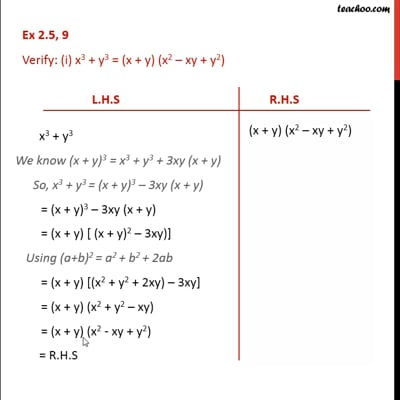Identity VIII

Chapter 2 Class 9 Polynomials (Term 2)
Concept wiseThis video is only available for Teachoo black users

### Transcript

Ex 2.5, 9 Verify: (i) x3 + y3 = (x + y) (x2 – xy + y2) L.H.S x3 + y3 We know (x + y)3 = x3 + y3 + 3xy (x + y) So, x3 + y3 = (x + y)3 – 3xy (x + y) = (x + y)3 – 3xy (x + y) = (x + y) [ (x + y)2 – 3xy)] Using (a+b)2 = a2 + b2 + 2ab = (x + y) [(x2 + y2 + 2xy) – 3xy] = (x + y) (x2 + y2 – xy) = (x + y) (x2 - xy + y2) = R.H.S R.H.S (x + y) (x2 – xy + y2) Hence proved